This would be a showcase of what kind of polling operations we can use in PyTorch.

%matplotlib inline
import matplotlib.pyplot as plt
import numpy as np
import os

import torch
import torch.nn as nn
import torch.nn.functional as F
from torch.utils.data import DataLoader, Dataset, TensorDataset

import torchvision
import torchvision.transforms as transforms

os.environ['KMP_DUPLICATE_LIB_OK']='True' #OpenMP
bs=512

t = transforms.Compose([
transforms.ToTensor(),
transforms.Normalize(mean=(0,), std=(1,))
]
)

batch_size=bs, drop_last=True, shuffle=True)
img = None
for (images,targets) in dl_train:
img = images
break

img = img.permute(1, 2, 0)#.numpy()
print(type(img),img.shape)
plt.imshow(img, cmap="gray")
plt.show()



Out: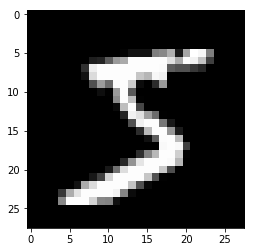We used the matplotlib.pyplot method imshow to show the single image from the MNIST dataset.

Now we will use pooling operations on that image.

img = img.reshape((1,1,28,28))
print("original image:", img.shape)
plt.imshow(img.squeeze(), cmap="gray")
plt.show()

mp = F.max_pool2d(img,(2,2))
print("max_pool2d result:", mp.shape)
plt.imshow(mp.squeeze(), cmap="gray")
plt.show()

ap = F.avg_pool2d(img, 2)
print("avg_pool2d result:", ap.shape)
plt.imshow(ap.squeeze(), cmap="gray")
plt.show()

aap = F.adaptive_avg_pool2d(img, 6)
plt.imshow(aap.squeeze(), cmap="gray")
plt.show()

amp = F.adaptive_max_pool2d(img, 6)
plt.imshow(amp.squeeze(), cmap="gray")
plt.show()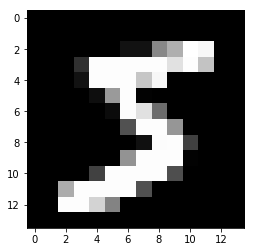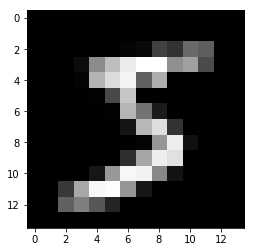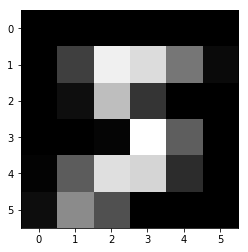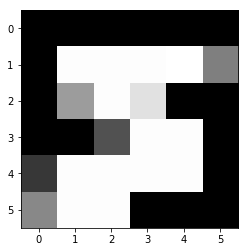In PyTorch we use pooling operations on tensors that represent a batch. This is why we used :

img = img.reshape((1,1,28,28))


The results will be:

max_pool2d result: torch.Size([1, 1, 14, 14])
avg_pool2d result: torch.Size([1, 1, 14, 14])
adaptive_avg_pool2d result: torch.Size([1, 1, 6, 6])
adaptive_max_pool2d result: torch.Size([1, 1, 6, 6])


As you may know, to plot the image inline we use %matplotlib inline and matplotlib.pyplot method imshow.

This method requires either the image is 2D, or it has the channel dimension at the very end, say:

• either [28,28]
• or [28,28, c]

where c is usually 1, or 3.